# Problem 53905. Easy Sequences 64: Base-14 Arithmetic Function

For an integer n, the base-14 arithmetic function,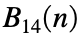, is defined as follows:
• Convert n to base-14, using the letters '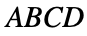' for digits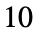to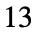.
• Replace the letters '' with the arithmetic operation symbols '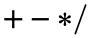', respectively.
• Remove any leading or trailing operation symbols.
• For consecutive operation symbols between two numeric symbols, retain only the first operation and remove all others.
• Evaluate the resulting expression in base-10.
• Return the evaluated expression as, rounded-off to the nearest integer.
• Finally, output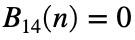ifcontains only "operation symbols", or if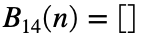, or if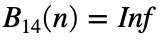, or if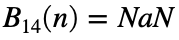.
For example if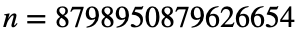: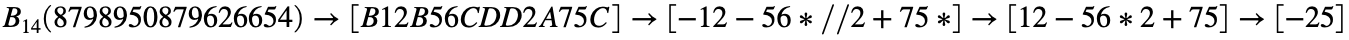Therefore: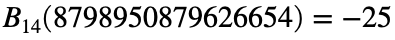.
Given a set positive integers N, find the integer x,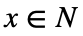, such that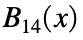is minimized. If there are more than one integers, x in N that minimizes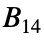, please output the largest of those x's.
For example for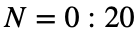:Therefore,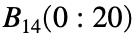is minimized when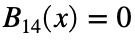, for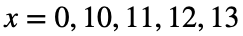. Since we are asked to select the largest minimizer, our program output should be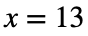.

### Solution Stats

85.71% Correct | 14.29% Incorrect
Last Solution submitted on Sep 15, 2023

### Community Treasure Hunt

Find the treasures in MATLAB Central and discover how the community can help you!

Start Hunting!